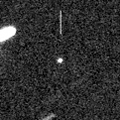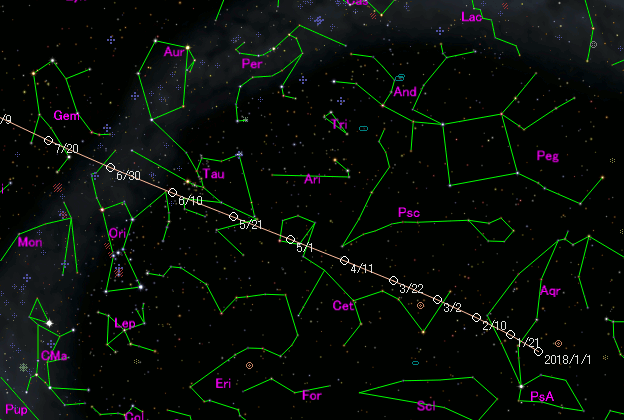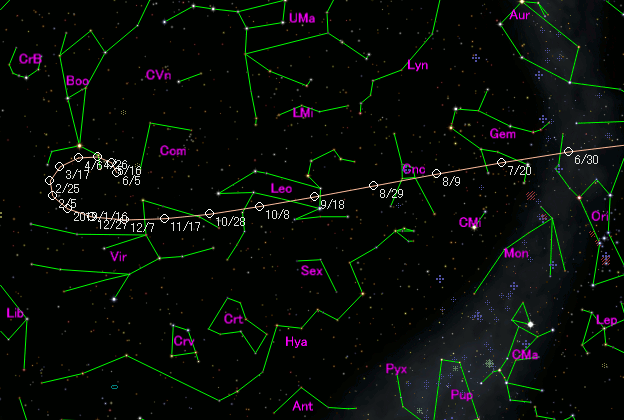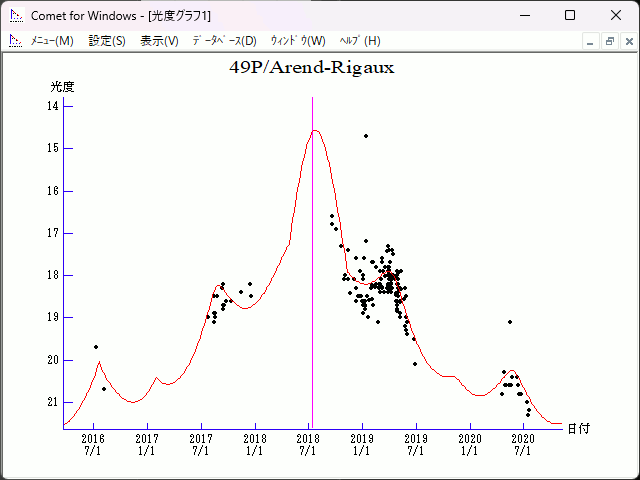# \$B%"%i%s(B-\$B%j%4!

49P/Arend-Rigaux (2018)###\$B###\$B50F;MWAG(B

```Epoch 2018 July 21.0 TT = JDT 2458320.5
T 2018 July 15.49797 TT                                 Rudenko
q   1.4296056            (2000.0)            P               Q
n   0.14618284     Peri.  332.88787     -0.05172985     -0.95687533
a   3.5689335      Node   118.82432     +0.96518837     -0.12139110
e   0.5994306      Incl.   19.04323     +0.25638921     +0.26392007
P   6.74
From 3418 observations 1963 Oct. 18-2020 July 20, mean residual 0".6.
Nongravitational parameters A1 = +0.02, A2 = -0.0005.
```

###\$B@1?^(B###\$B8wEYJQ2=(B

```        H = 13.5  G = 0.15                       [   , -80]  (              \$B!A(B2018\$BG/(B 4\$B7n(B26\$BF|(B)
m1 = 8.0 + 5 log\$B&\$(B + 30.0 log r(t - 10)  [-80, 117]  (2018\$BG/(B 4\$B7n(B26\$BF|!A(B2018\$BG/(B11\$B7n(B 9\$BF|(B)
H = 13.5  G = 0.15                       [117,    ]  (2018\$BG/(B11\$B7n(B 9\$BF|!A(B              )
```##### \$B50F;MWAG\$O(BM.P.E.C. 2020-P19\$B\$K7G:\\$5\$l\$?\$b\$N\$G\$9!#(B \$B@1?^\$O%9%F%i%J%S%2!<%?(B Ver.8 (\$B%"%9%H%m%"!<%D(B) \$B\$G:n@.\$7\$?\$b\$N\$G\$9!#(B \$B8wEY%0%i%U\$O(BComet for Windows\$B\$G:n@.\$7\$?\$b\$N\$G\$9!#(B